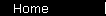R-Squared

Statistical measure of how well a regression line approximates real data points; an r-squared of 1.0 (100%) indicates a perfect fit. The formula for r is:
r(X,Y)   =   [ Cov(X,Y) ] / [ StdDev(X) x StdDev(Y) ]

In finance, r-squared measures how well the Capital Asset Pricing Model predicts the actual performance of an investment or portfolio.

See the main article on CAPM regression.

 home  |  glossary  |  calculator  |  about us  |  books